International
Tables for
Crystallography
Volume D
Physical properties of crystals
Edited by A. Authier

International Tables for Crystallography (2006). Vol. D, ch. 1.2, pp. 34-35

## Section 1.2.1. Introduction

T. Janssena*

aInstitute for Theoretical Physics, University of Nijmegen, 6524 ED Nijmegen, The Netherlands
Correspondence e-mail: ted@sci.kun.nl

### 1.2.1. Introduction

| top | pdf |

Symmetry arguments play an important role in science. Often one can use them in a heuristic way, but the correct formulation is in terms of group theory. This remark is in fact superfluous for crystallographers, who are used to point groups and space groups as they occur in the description of structures. However, besides these structural problems there are many others where group theory may play a role. A central role in this context is played by representation theory, which treats the action of a group on physical quantities, and usually this is done in terms of linear transformations, although nonlinear representations may also occur.

To start with an example, consider a spin system, an arrangement of spins on sites with a certain symmetry, for example space-group symmetry. The elements of the space group map the sites onto other sites, but at the same time the spins are rotated or transformed otherwise in a well defined fashion. The spins can be seen as elements of a vector space (spin space) and the transformation in this space is an image of the space-group element. In a similar way, all symmetric tensors of rank 2 form a vector space, because one can add them and multiply them by a real factor. A linear change of coordinates changes the vectors, and the transformations in the space of tensors are the image of the coordinate transformations. Probably the most important use of such representations is in quantum mechanics, where transformations in coordinate space are mapped onto linear transformations in the quantum mechanical space of state vectors.

To see the relation between groups of transformations and the use of their representations in physics, consider a tensor which transforms under a certain point group. Let us take a symmetric rank 2 tensorin three dimensions. We take as example the point group 222. From Section 1.1.3.2one knows how such a tensor transforms: it transforms into a tensor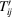according to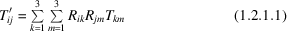for all orthogonal transformations R in the group 222. This action of the point group 222 is obviously a linear one: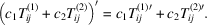The transformations on the tensors really form an image of the group, because if one writes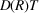for, one has for two elements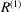and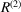the relation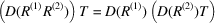or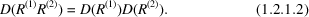This property is said to define a (linear) representation. Because of the representation property, it is sufficient to know how the tensor transforms under the generators of a group. In our example, one could be interested in symmetric tensors that are invariant under the group 222. Then it is sufficient to consider the rotations over 180° along the x and y axes. If the point group is a symmetry group of the system, a tensor describing the relation between two physical quantities should remain the same. For invariant tensors one has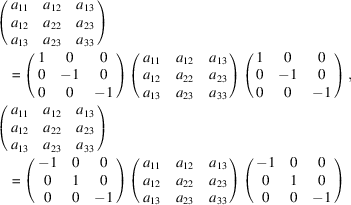and the solution of these equations is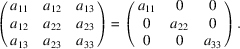The matrices of rank 2 form a nine-dimensional vector space. The rotation over 180° around the x axis can also be written as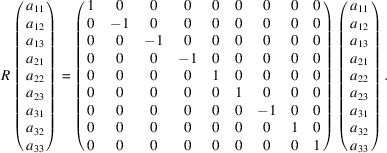This nine-dimensional matrix together with the one corresponding to a rotation along the y axis generate a representation of the group 222 in the nine-dimensional space of three-dimensional rank 2 tensors. The invariant tensors form the subspace (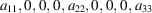). In this simple case, group theory is barely needed. However, in more complex situations, the calculations may become quite cumbersome without group theory. Moreover, group theory may give a wealth of other information, such as selection rules and orthogonality relations, that can be obtained only with much effort without group theory, or in particular representation theory. Tables of tensor properties, and irreducible representations of point and space groups, have been in use for a long time. For point groups see, for example, Butler (1981) and Altmann & Herzig (1994); for space groups, see Miller & Love (1967), Kovalev (1987) and Stokes & Hatch (1988).

In the following, we shall discuss the representation theory of crystallographic groups. We shall adopt a slightly abstract language, which has the advantage of conciseness and generality, but we shall consider examples of the most important notions. Another point that could give rise to some problems is the fact that we shall consider in part the theory for crystallographic groups in arbitrary dimension. Of course, physics occurs in three-dimensional space, but often it is useful to see what is general and what is special for one, two or three dimensions. In Section 1.2.2, the point groups are discussed, together with their representations. In Section 1.2.3, the same is done for space groups. Tensors for point and space groups are then treated in terms of representation theory in Section 1.2.4. Besides transformations in space, transformations involving time reversal are important as well. They are discussed in Section 1.2.5. Information on crystallographic groups and their representations is presented in tabular form in Section 1.2.6. This section can be consulted independently.

### References

Altmann, S. L. & Herzig, P. (1994). Point-group theory tables. Oxford: Clarendon Press.
Butler, P. H. (1981). Point group symmetry applications. New York & London: Plenum Press.
Kovalev, O. V. (1987). Representations of the crystallographic space groups. New York: Gordon and Breach.
Miller, S. C. & Love, W. F. (1967). Tables of irreducible representations of space groups and co-representations of magnetic space groups. Boulder, Colorado: Pruett Press.
Stokes, H. T. & Hatch, D. M. (1988). Isotropy subgroups of the 230 crystallographic space groups. Singapore: World Scientific.